# HSRL measurement principles and measured parameters

In order to make reliable measurements of the extinction profile the HSRL measures two signals which can be processed to yield separate lidar returns for aerosol and molecular scattering. This separation is possible because the spectrum of the molecular lidar return is Doppler broadened by the thermal motion of the molecules while the slow moving aerosol particles generate negligible spectral broadening. The molecular scattering cross section is a function of molecular density and can be calculated from Rayleigh scattering theory and an independently measured temperature profile. The HSRL then uses molecular scattering as a calibration target which is available at each point in the lidar return.

Two lidar returns are derived; these are given by the following equations for the signal power received from molecular scattering,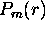, and aerosol scattering,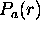: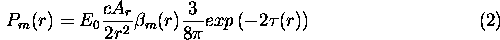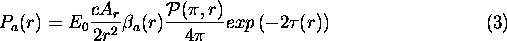Equation 3 contains two unknowns: the optical depth between the lidar and the scattering volume,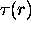, and the molecular scattering cross section,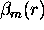. Molecular scattering is described by the Rayleigh scattering equation and is directly proportional to atmospheric density,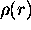: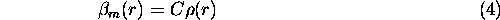Thus if we define a range-squared, energy corrected molecular lidar return: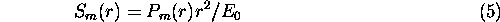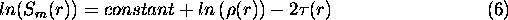and the optical depth between any two ranges, r and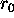, is given by: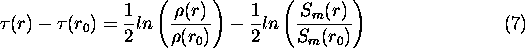The average extinction cross section in a layer betweenand r can be computed from equation 8: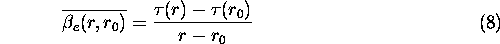Notice that this equation can be used to generate vertical profiles of the extinction cross section; however, the vertical resolution will be dictated by the minimum layer thickness required to detect differences in optical depth.

A ratio computed between the aerosol lidar return and the molecular return provides the lidar backscatter ratio,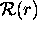: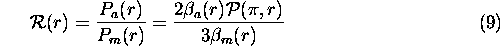The lidar backscatter ratio and the molecular backscatter cross section can be used to calculate the aerosol backscatter cross section: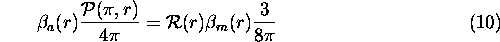and the ratio of the layer average aerosol backscatter cross section to the layer average aerosol extinction cross section provides the average backscatter phase function between r and rtimes the single scatter albedo, a(r):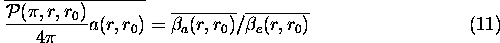For water and ice clouds and most aerosols the single scatter albedo at the 532 nm wavelength of the HSRL is very close to unity; thus equation 10 provides the backscatter phase function.

Depolarization measurements allow discrimination between water and ice clouds. The linear depolarization ratio can be given by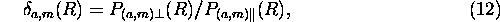where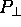and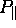are the signals incident on the receiver with polarization perpendicular and parallel to the receiver polarization axis, respectively. Separate measurements of aerosol and molecular depolarization are acquired.